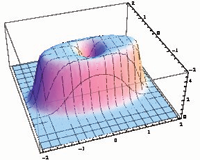April 2011
Mon Tue Wed Thu Fri Sat Sun
« Mar   May »
123
45678910
11121314151617
18192021222324
252627282930

## Non-Hermitian extensions of Wishart random matrix ensembles

Gernot AkemannWe briefly review the solution of three ensembles of non-Hermitian random matrices generalizing the Wishart-Laguerre (also called chiral) ensembles. These generalizations are realized as Gaussian two-matrix models, where the complex eigenvalues of the product of the two independent rectangular matrices are sought, with the matrix elements of both matrices being either real, complex or quaternion real. We also present the more general case depending on a non-Hermiticity parameter, that allows us to interpolate between the corresponding three Hermitian Wishart ensembles with real eigenvalues and the maximally non-Hermitian case. All three symmetry classes are explicitly solved for finite matrix size NxM for all complex eigenvalue correlations functions (and real or mixed correlations for real matrix elements). These are given in terms of the corresponding kernels built from orthogonal or skew-orthogonal Laguerre polynomials in the complex plane. We then present the corresponding three Bessel kernels in the complex plane in the microscopic large-N scaling limit at the origin, both at weak and strong non-Hermiticity with M-N greater or equal to 0 fixed.

http://arxiv.org/abs/1104.5203
Mathematical Physics (math-ph); Statistical Mechanics (cond-mat.stat-mech)